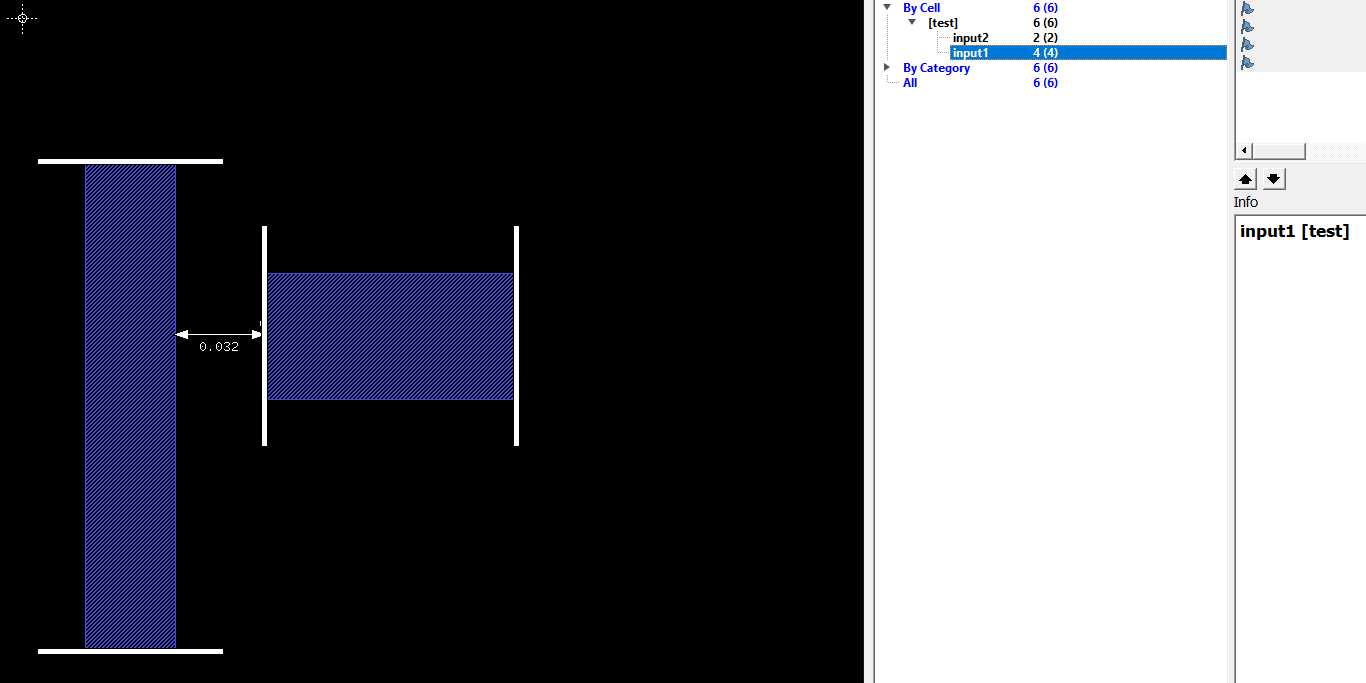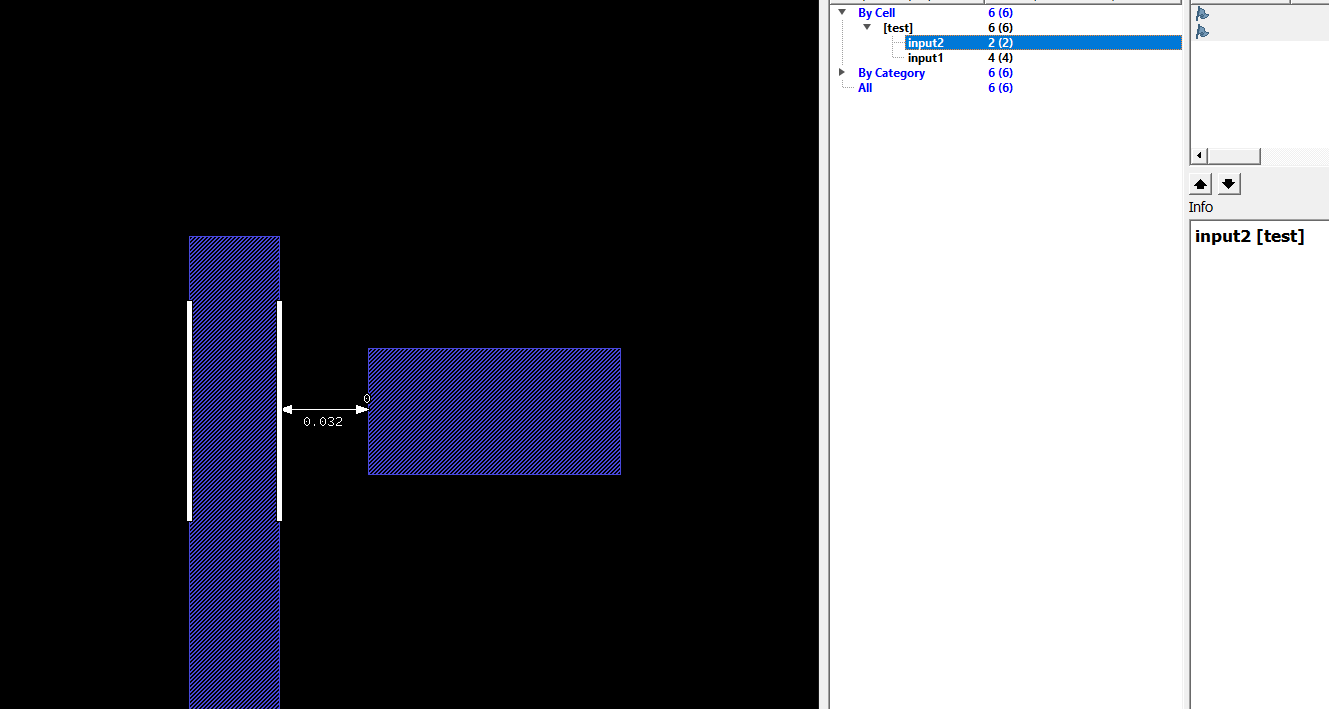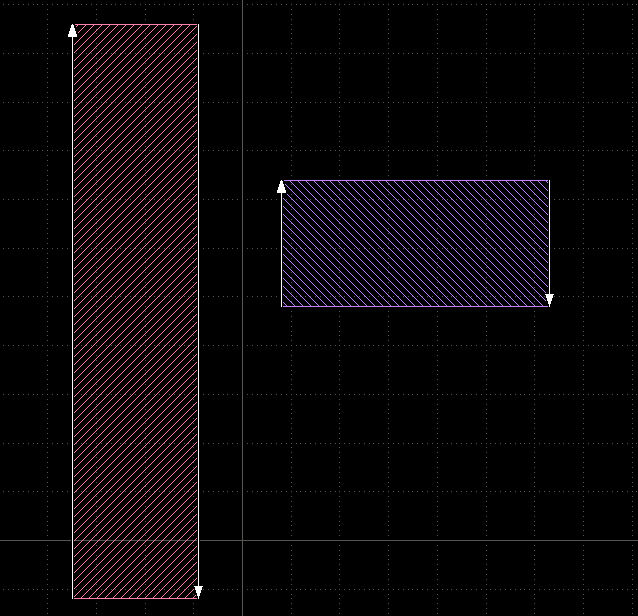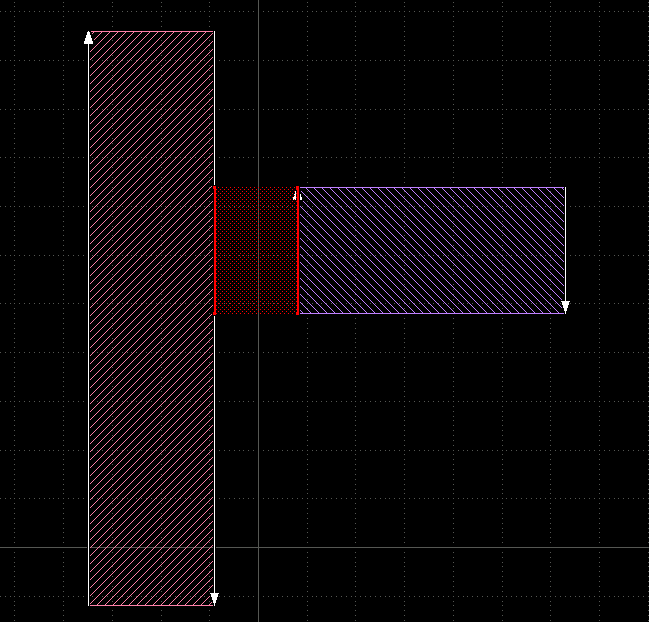# separation edges to edges issue

Hi @Matthias ,
I tried to use "separation" drc for edges but result got is unexpected( not flagged):

``````input2.output("input2")
input1.output("input1")
input1.separation(input2, 0.081,projection).output("space less 81nm")
``````

In this case i expected drc will be flagged.
Can you help me show my mistake here?.Thank you so much!
dai

• Hello @dai,

There is one important thing about edges: their orientation matters.

I have prepared a sample:

This is the code:

``````report("Discussion 2102")

input1 = input(1, 0)
input2 = input(2, 0)

vedges1 = input1.edges.with_angle(90.0)
vedges2 = input2.edges.with_angle(90.0)

vedges1.output("vedges1")
vedges2.output("vedges2")
``````

with this result (red is 1/0, blue is 2/0):I have marked the edges with arrows to indicate their orientation.

Only the shown orientation will give you the desired separation result. So when I use:

``````vedges1.separation(vedges2, 0.2.um, projection).output("separation < 0.2µm")
``````

I get this result:The idea is that edges basically carry the information about the features they are enclosing. As KLayout uses clockwise polygon orientation, "right" of the edge is "inside" and "left" is outside. The separation check is actually not a separation check between edges, but a separation check between the polygons they were originally enclosing.

There are other variants for edge-to-edge checks too: width, enclosing and enclosed. All of them apply to a different relative orientation of the involved edges.

But I understand it's only about distance of unoriented edges in your case, or can you (by the way you generate the edges) impose a certain relative orientation?

Maybe I should add a "non-orientated separation" feature too.

Matthias

• Thank you, @Matthias
I have extended the edge to tiny polygon(i know it is not good solution but it can resolve my issue) and apply space DRC to them

But I understand it's only about distance of unoriented edges in your case, or can you (by the way you generate the edges) impose a certain relative orientation?

Here is the way i created the edges,

report("DRC")
metal = input(31,0)
line_end_metal_90 = metal.corners(90, as_edge_pairs).edges
line_end_metal_270 = metal.corners(90..270, as_edge_pairs).edges
line_end_metal = line_end_metal_270 - line_end_metal_90
G0S_81 = 81.nm
END_LINE_46 = 46.nm
PRL_NEG_16 = 16.nm
end_line_less_46 = line_end_metal.with_length(nil, END_LINE_46)
ext = end_line_less_46.extended(PRL_NEG_16,PRL_NEG_16,G0S_81,0)
ext.output("ext")
input1 = ext.edges.interacting(end_line_less_46)
input2 = (metal.edges.and(ext) - end_line_less_46)
input2.output("input2")
input1.output("input1")
input1.separation(input2,81.nm,projection).output("ERR")

i also attached the layout here.

thanks
dai

• I see. I admit I cannot predict the orientation of the edges after they go through these operations. There are rules, but one gets lost after a couple of operations.

Your solution is a fair workaround as of now. I think this is motivation for a non-oriented space or separation check between edges. I have created a ticket for this feature: https://github.com/KLayout/klayout/issues/1101

Matthias

• Thank you, @Matthias

dai